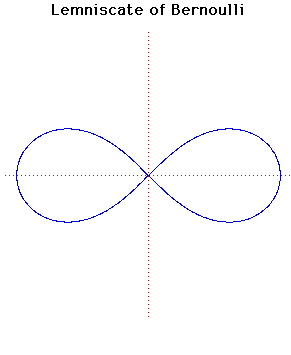# Curves

### Lemniscate of BernoulliCartesian equation:
$(x^{2} + y^{2})^{2} = a^{2}(x^{2} - y^{2})$
Polar equation:
$r^{2} = a^{2}\cos(2 \theta )$

### Description

In 1694 Jacob Bernoulli published an article in Acta Eruditorumon a curve
shaped like a figure 8, or a knot, or the bow of a ribbon
which he called by the Latin word lemniscus ('a pendant ribbon'). Jacob Bernoulli was not aware that the curve he was describing was a special case of a Cassinian Oval which had been described by Cassini in 1680.

The general properties of the lemniscate were discovered by Giovanni Fagnano in 1750. Euler's investigations of the length of arc of the curve (1751) led to later work on elliptic functions.

Inverting the lemniscate in a circle centred at the origin and touching the lemniscate where it crosses the $x$-axis produces a rectangular hyperbola.

The bipolar equation of the lemniscate is $rr' = a^{2}/2$.

Other Web site:

Xah Lee

### Associated Curves

Definitions of the Associated curves